Ch 7. Stress Analysis Multimedia Engineering Mechanics PlaneStress PrincipalStresses Mohr's Circlefor Stress Failure PressureVessels
 Chapter 1. Stress/Strain 2. Torsion 3. Beam Shr/Moment 4. Beam Stresses 5. Beam Deflections 6. Beam-Advanced 7. Stress Analysis 8. Strain Analysis 9. Columns Appendix Basic Math Units Basic Equations Sections Material Properties Structural Shapes Beam Equations Search eBooks Dynamics Fluids Math Mechanics Statics Thermodynamics Author(s): Kurt Gramoll ©Kurt GramollMECHANICS - THEORY

Failure Thoeries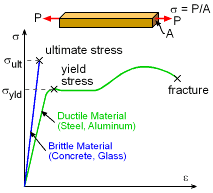Uniaxial Stress-Strain Curve with
Yield Stress and Ultimate Stress

If all structures where loaded in only one direction, it would be easy to predict failure. All that would be needed was a single uniaxial test to find the yield stress and ultimate stress levels. If it is a brittle material, then the ultimate stress will determine failure. For ductile material, failure is assumed to be when the material starts to yield and permanently deform.

However, when a structure has multiple stresses at a given local (σx, σy and τxy for 2D as discussed in Stresses at a Point section), then the interaction between those stresses may effect the final failure. This section presents three basic failure theories that can be used for different types of materials to help predict failure when multiple stresses are applied.

For simplification, all theories are based on principal stresses1, σ2) which can be determined from any (σx, σy and τxy) stress state. This removes the shear stress terms since the shear stress is zero at the principal directions. Using principal stresses does not change the results from the failure theories.

Maximum Normal Stress Theory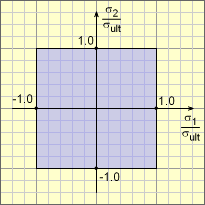Maximum Normal Stress Criterion
(Blue Regoin is Safe)

The simpliest theory ignores any interaction between the normal principal stresses, and assumes that failure occurs when either of the normal stresses exceed the ultimate stress. This is written as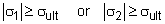This can be visualized on a plot by normalizing the two normal stress as σ1ult and σ2ult. This gives a square region where the stress state is safe. Outside the region is failure.

This failure criteria is really good for brittle materials and should not used for ductile material like steel, aluminum, and plastics.

Maximum Shear Stress Theory
(Tresca's Yield Criterion)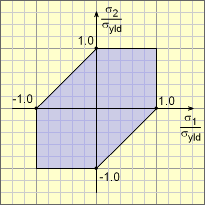Maximum Shear Stress Criterion
or Tresca's Yield Criterion
(Blue Regoin is Safe)

The maximum shear stress assumes failure occurs when the maximum shear stress exceeds the shear stress in a simple uniaxial test. In a unixial test, the principal stresses are σ1 = σx (axial direction) and σ2 = 0 (transverse to axial direction). Using the stress transformation equations, the maximum shear stress for this stress state is

τmax = (σx - σy)/2 = σx/2 = σyld/2

Thus, for any combination of loading for σ1 and σ2, the shear stress cannot exceed = σyld/2. This condition gives three seperate possible situations that need to checked,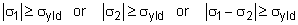This criteria is actually fairly accurate for ductile materials like steel, alumunim and plastics. The difficulty is that three conditions need to be checked.

Maximum Distortion Energy Theory
(von Mises' Yield Criterion)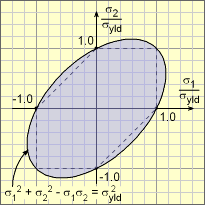Maximum Distortion Energy Criterion
or von Mises' Yield Criterion
(Blue Regoin is Safe)

The third theory looks at the total energy at failure and compares that with the total energy in a unixial test at failure. Any elastic member under load acts like a spring and stores energy. This is commonly called distortational energy and can be calculated as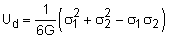The G is the shear modulus. The distortion energy for a general stress state can be compared to distortion energy for a uniaxial test that fails at σx = σ1 = σyld. This gives,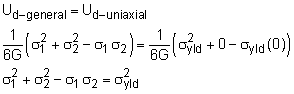Thus the general criterion for failure would be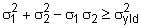This criteria is especially useful since it is a single equation. It is also accurate for ductile materials. The shape of the region is an ellispe that is rotated 45 degrees.

Maximum Distortion Energy Theory (3D)

For 3D stress state, a similar calculation can be done based on the three principal stresses, σ1, σ2 and σ3.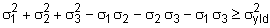Practice Homework and Test problems now available in the 'Eng Mechanics' mobile app
Includes over 400 problems with complete detailed solutions.
Available now at the Google Play Store and Apple App Store.